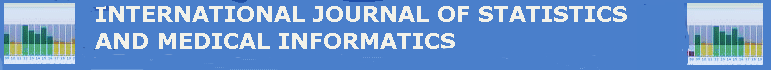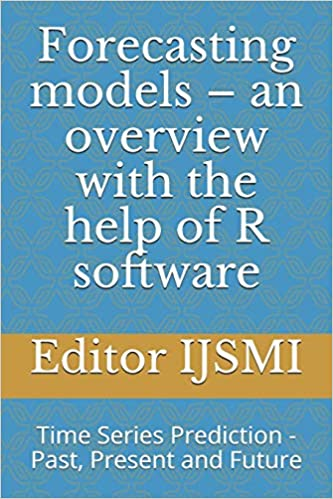Forecasting models - an overview with the help of R software : Time series - Past ,Present and Future

Preface

Forecasting models involves predicting the future values of a particular series of data which is mainly based on the time domain. Forecasting models are widely used in the fields such as financial markets, demand for a product and disease outbreak. The objective of the forecasting model is to reduce the error in the forecasting.

Most of the Forecasting models are based on time series, a statistical concept which involves Moving Averages, Auto Regressive Integrated Moving Averages (ARIMA), Exponential smoothing and Generalized Auto Regressive Conditional Heteroscedastic (GARCH) Models. Forecasting models which we deal in this book will be explorative forecasting models which take into account the past data to predict the future values.

Current day forecasting models uses advanced techniques such as Machine Learning and Deep Learning Algorithms which are more robust and can handle high volume of data.

This book starts with the overview of forecasting and time series concepts and moves on to build forecasting models using different time series models. Examples related to forecasting models which are built based on Machine learning also covered. The book uses R statistical software package, an open source statistical package to build the forecasting models.

Purchase from Amazon

Hardcover
(Price-\$32)

Purchase from Amazon

e-Book
(Price - \$9.99)

Paperback
(Price-\$27.50)

;